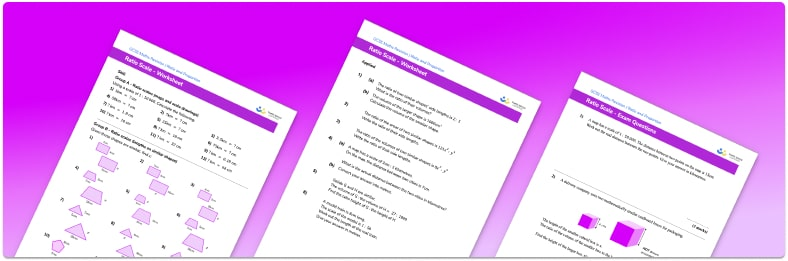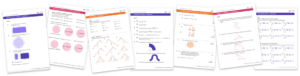# Ratio And Scale Worksheet• Section 1 of the ratio and scale worksheet contains 36 skills-based ratio and scale questions, in 3 groups to support differentiation
• Section 2 contains 4 applied ratio and scale questions with a mix of worded problems and deeper problem solving questions
• Section 3 contains 4 foundation and higher level GCSE exam style ratio and scale questions
• Answers and a mark scheme for all ratio and scale questions are provided
• Questions follow variation theory with plenty of opportunities for students to work independently at their own level
• All questions created by fully qualified expert secondary maths teachers
• Suitable for GCSE maths revision for AQA, OCR and Edexcel exam boards

• This field is for validation purposes and should be left unchanged.

You can unsubscribe at any time (each email we send will contain an easy way to unsubscribe). To find out more about how we use your data, see our privacy policy.

### Ratio and scale at a glance

Ratio is often used to give a scale between two different things. Real life examples of this  include a map scale, for example 1:25000 , a scale model of an object such as a model train in the scale 1:160 or a scale drawing.

The scale factor between two things is often given as a ratio in the form 1:n where n can be an integer, fraction, or a decimal. We can use the given ratio to calculate lengths of one thing based on the other. If the ratio of two items A:B is in the simplest form of 1:n then B is n times bigger (or smaller) than A .

For example, if a map has a scale of 1:100000 then two points which are 4cm apart on the map will be  4100000=400000cm  or  4km apart in real life.

Looking forward, students can then progress to additional ratio worksheets and other ratio and proportion worksheets, for example a speed distance time worksheet or a direct proportion worksheet.For more teaching and learning support on Ratio and Proportion our GCSE maths lessons provide step by step support for all GCSE maths concepts.

## Do you have KS4 students who need more focused attention to succeed at GCSE?There will be students in your class who require individual attention to help them succeed in their maths GCSEs. In a class of 30, it’s not always easy to provide.

Help your students feel confident with exam-style questions and the strategies they’ll need to answer them correctly with our dedicated GCSE maths revision programme.

Lessons are selected to provide support where each student needs it most, and specially-trained GCSE maths tutors adapt the pitch and pace of each lesson. This ensures a personalised revision programme that raises grades and boosts confidence.

Find out more